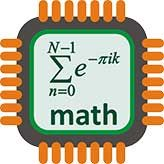# Chunking: Division AlgorithmDivision is can be a hard concept for some students to grasp. However, using this interactive website to explore how to chunk division algorithms could be a solution that eases the process of dividing. When given a problem, students use the "power of ten" buttons to multiply the given dividend to get as close to that number without going over. Then they subtract and click the equal button to see what number is left. By continuing this process until they reach a number that can not be subtracted further, students will be able to see a relationship between division and subtraction, making the whole process more clear. Students will gain confidence using chunking to divide algorithms that normally seem overwhelming.

courtesy of Knovation